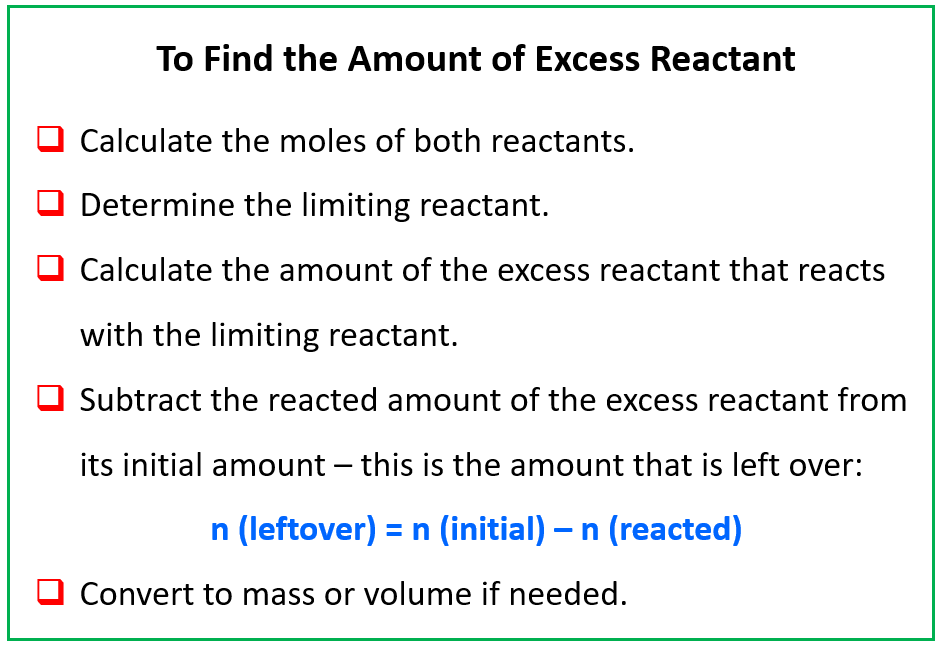## General Chemistry

When two substances are reacted in a nonstoichiometric ratio, one of the is going to be the limiting reactant (LR), and the other is the excess reactant. The limiting reactant is the one that runs out first, and therefore, defines the amount of the products that are formed. Remember, we cannot determine the limiting reactant by simply comparing the amounts of the reactants. You can review the concept of limiting reactant here if you need to.

Now, when the limiting reactant is finished, there is still going to be some of the excess reactant left over.

So, how do we calculate the amount of the excess reactant once the reaction is finished?

To do this, we need to follow all the steps we have covered in the stoichiometry of chemical reactions such as calculating the moles of the reactants and determining the limiting reactant. Once we know the limiting reactant, we calculate the moles of the excess reactant that reacts with it according to the stoichiometric ratio. In the end, we subtract the amount of the excess reactant that reacted with the limiting reactant from its initial amount.

For example, consider the reaction between MnO2 and HCl:

MnO2 + 4HCl → MnCl2 + Cl2 + 2H2O

How many grams of the excess reactant is left over when 165 g of MnO2 is added to a solution containing 94.2 g of HCl?

The amounts of both reactants are given in grams, so we need to first calculate the moles and determine the LR.

The moles of MnO2 and HCl are:

${\rm{n}}\;{\rm{(Mn}}{{\rm{O}}_{\rm{2}}}{\rm{)}}\;{\rm{ = }}\;{\rm{165}}\;{\rm{g}}\;{\rm{ \times }}\;\frac{{{\rm{1}}\;{\rm{mol}}}}{{{\rm{86}}{\rm{.9}}\;{\rm{g}}}}\;{\rm{ = }}\;{\rm{1}}{\rm{.90}}\;{\rm{mol}}$

${\rm{n}}\;{\rm{(HCl)}}\;{\rm{ = }}\;{\rm{94}}{\rm{.2}}\;{\rm{g}}\;{\rm{ \times }}\;\frac{{{\rm{1}}\;{\rm{mol}}}}{{{\rm{36}}{\rm{.5}}\;{\rm{g}}}}\;{\rm{ = }}\;{\rm{2}}{\rm{.58}}\;{\rm{mol}}$

Next, we calculate how much MnCl2 can be formed from each reactant in order to determine the limiting reactant. The one that gives less amount of product is the limiting reactant. We can do this based on any of the products as long as we keep it consistent and use the same product for both reactants.

${\rm{n}}\;\left( {{\rm{MnC}}{{\rm{l}}_{\rm{2}}}} \right)\;{\rm{ = }}\;{\rm{1}}{\rm{.90 }}\cancel{{{\rm{mol}}\;{\rm{Mn}}{{\rm{O}}_{\rm{2}}}}}\;{\rm{ \times }}\;\frac{{{\rm{1}}\;{\rm{mol}}\;{\rm{MnC}}{{\rm{l}}_{\rm{2}}}}}{{{\rm{1}}\;\cancel{{{\rm{mol}}\;{\rm{Mn}}{{\rm{O}}_{\rm{2}}}}}}}\;{\rm{ = }}\;{\rm{1}}{\rm{.90}}\;{\rm{mol}}$

${\rm{n}}\;\left( {{\rm{MnC}}{{\rm{l}}_{\rm{2}}}} \right)\;{\rm{ = }}\;{\rm{2}}{\rm{.58 }}\cancel{{{\rm{mol}}\;{\rm{HCl}}}}\;{\rm{ \times }}\;\frac{{{\rm{1}}\;{\rm{mol}}\;{\rm{MnC}}{{\rm{l}}_{\rm{2}}}}}{{{\rm{4}}\;\cancel{{{\rm{mol}}\;{\rm{HCl}}}}}}\;{\rm{ = }}\;{\rm{0}}{\rm{.645}}\;{\rm{mol}}$

HCl gives less product so, it is the LR. The 0.645 mol MnCl2 is the theoretical yield of the reaction, however, our focus here is to determine the amount of MnO2 that is left after the reaction is complete.

Now, let’s calculate how many moles of MnO2 will react with the 2.58 mol of HCl:

${\rm{n}}\;{\rm{(Mn}}{{\rm{O}}_{\rm{2}}}\,{\rm{reacted)}}\;{\rm{ = }}\;{\rm{2}}{\rm{.58}}\,{\rm{mol HCl}}\;{\rm{ \times }}\;\frac{{{\rm{1}}\;{\rm{mol}}\,{\rm{Mn}}{{\rm{O}}_{\rm{2}}}}}{{{\rm{4}}\;{\rm{mol}}\,{\rm{HCl}}}}\;{\rm{ = }}\;{\rm{0}}{\rm{.645}}\;{\rm{mol}}$

At his point, we need to calculate how many moles of MnO2 is left over knowing that initially there were 1.90 mol, and 0.645 of hat had reacted with the HCl.

Subtracting these, we get that there is 1.255 mol MnO2 left:

n (excess MnO2) = n (initial MnO2) – n (reacted MnO2)

n (excess MnO2) = 1.90 – 0.645 = 1.255 mol

In the end, we only need to convert the moles to the mass of MnO2.

${\rm{m}}\;{\rm{(Mn}}{{\rm{O}}_{\rm{2}}}{\rm{)}}\;{\rm{ = }}\;{\rm{1}}{\rm{.255}}\;{\rm{mol}}\;{\rm{ \times }}\;\frac{{{\rm{86}}{\rm{.9}}\;{\rm{g}}}}{{{\rm{1}}\;{\rm{mol}}}}\;{\rm{ = }}\;{\rm{109}}\;{\rm{g}}$

Let’s do another example.

How many grams of the excess reactant is left over if 130.5 g Cl2 is reacted with 56.4 g P4 according to the following equation?

6Cl2(g) + P4(s) → 4PCl3(l)

When the amount of both reactants is given, you must determine the LR and then calculate the amount of excess reactant. So, the first step is to determine the moles of the reactants:

${\rm{n}}\;{\rm{(C}}{{\rm{l}}_{\rm{2}}}{\rm{)}}\;{\rm{ = }}\;130.5\;{\rm{g}}\;{\rm{ \times }}\;\frac{{{\rm{1}}\;{\rm{mol}}}}{{{\rm{70}}{\rm{.9}}\;{\rm{g}}}}\;{\rm{ = }}\;{\rm{1}}{\rm{.84}}\;{\rm{mol}}$

${\rm{n}}\;{\rm{(}}{{\rm{P}}_{\rm{4}}}{\rm{)}}\;{\rm{ = }}\;{\rm{56}}{\rm{.4}}\;{\rm{g}}\;{\rm{ \times }}\;\frac{{{\rm{1}}\;{\rm{mol}}}}{{{\rm{123}}{\rm{.9}}\;{\rm{g}}}}\;{\rm{ = }}\;{\rm{0}}{\rm{.455}}\;{\rm{mol}}$

Next, we calculate, for example, how much PCl3 can be formed from each reactant:

${\rm{n}}\;\left( {{\rm{PC}}{{\rm{l}}_{\rm{3}}}} \right)\;{\rm{ = }}\;{\rm{1}}{\rm{.84 }}\cancel{{{\rm{mol}}\;{\rm{C}}{{\rm{l}}_{\rm{2}}}}}\;{\rm{ \times }}\;\frac{{{\rm{4}}\;{\rm{mol}}\;{\rm{PC}}{{\rm{l}}_{\rm{3}}}}}{{{\rm{6}}\;\cancel{{{\rm{mol}}\;{\rm{C}}{{\rm{l}}_{\rm{2}}}}}}}\;{\rm{ = }}\;{\rm{1}}{\rm{.23}}\;{\rm{mol}}$

${\rm{n}}\;\left( {{\rm{PC}}{{\rm{l}}_{\rm{3}}}} \right)\;{\rm{ = }}\;{\rm{0}}{\rm{.455 }}\cancel{{{\rm{mol}}\;{{\rm{P}}_{\rm{4}}}}}\;{\rm{ \times }}\;\frac{{{\rm{4}}\;{\rm{mol}}\;{\rm{PC}}{{\rm{l}}_{\rm{3}}}}}{{{\rm{1}}\;\cancel{{{\rm{mol}}\;{{\rm{P}}_{\rm{4}}}}}}}\;{\rm{ = }}\;{\rm{1}}{\rm{.82}}\;{\rm{mol}}$

Cl2 gives less PCl3 and therefore, it is the LR. Now, to calculate how many moles, out of the initial 0.455 moles of the P4, will react with 1.84 mol of Cl2, we do a mole-ratio calculation:

${\rm{n}}\;\left( {{{\rm{P}}_{\rm{4}}}\;{\rm{reacted}}} \right)\;{\rm{ = }}\;{\rm{1}}{\rm{.84 }}\cancel{{{\rm{mol}}\;{\rm{C}}{{\rm{l}}_{\rm{2}}}}}\;{\rm{ \times }}\;\frac{{{\rm{1}}\;{\rm{mol}}\;{{\rm{P}}_{\rm{4}}}}}{{{\rm{6}}\;\cancel{{{\rm{mol}}\;{\rm{C}}{{\rm{l}}_{\rm{2}}}}}}}\;{\rm{ = }}\;{\rm{0}}{\rm{.307}}\;{\rm{mol}}$

At his point, we need to calculate how many moles of P4 are left over knowing that initially there was 0.455 mol, and 0.307 of that had reacted with the Cl2.

Subtracting these, we get that there is 0.148 mol P4 left:

n (excess P4) = n (initial P4) – n (reacted P4)

n (excess P4) = 0.455 – 0.307 = 0.148 mol P4 left over

In the end, we only need to convert the moles to the mass of P4.

${\rm{m}}\;{\rm{(}}{{\rm{P}}_{\rm{4}}}{\rm{)}}\;{\rm{ = }}\;{\rm{0}}{\rm{.148}}\;{\rm{mol}}\;{\rm{ \times }}\;\frac{{{\rm{124}}\;{\rm{g}}}}{{{\rm{1}}\;{\rm{mol}}}}\;{\rm{ = }}\;{\rm{18}}{\rm{.4}}\;{\rm{g}}$

To summarize, when calculating the amount of excess reactant left over after the reaction, follow these steps:Check Also

1686019900